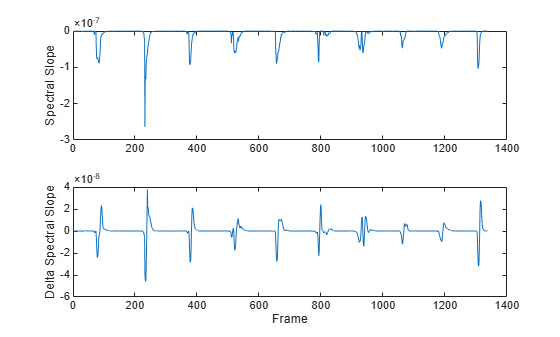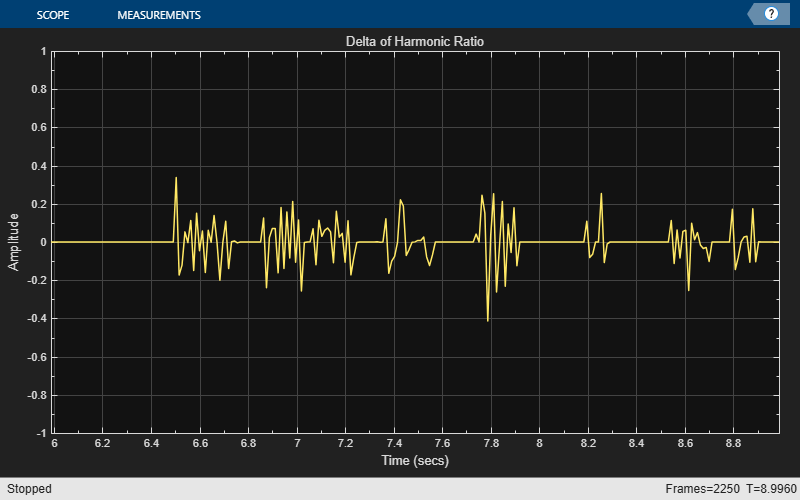audioDelta

Compute delta features

Description

example

delta = audioDelta(x) returns the delta features of input x. Columns of x are treated as independent channels.

example

delta = audioDelta(x,deltaWindowLength) specifies the delta window length.

example

delta = audioDelta(x,deltaWindowLength,initialCondition) specifies the initial condition of the filter.

example

[delta,finalCondition] = audioDelta(x,___) also returns the final condition of the filter.

Examples

collapse all

Create an audioFeatureExtractor object to extract some spectral features over time from the audio. Call extract to extract the audio features.

afe = audioFeatureExtractor('SampleRate',fs, ...
'spectralCentroid',true, ...
'spectralSlope',true);

audioFeatures = extract(afe,audioIn);

Call audioDelta to approximate the first derivative of the spectral features over time.

deltaAudioFeatures = audioDelta(audioFeatures);

Plot the spectral features and the delta of the spectral features.

map = info(afe);
tiledlayout(2,1)
nexttile
plot(audioFeatures(:,map.spectralCentroid))
ylabel('Spectral Centroid')
nexttile
plot(deltaAudioFeatures(:,map.spectralCentroid))
ylabel('Delta Spectral Centroid')
xlabel('Frame')tiledlayout(2,1)
nexttile
plot(audioFeatures(:,map.spectralSlope))
ylabel('Spectral Slope')
nexttile
plot(deltaAudioFeatures(:,map.spectralSlope))
ylabel('Delta Spectral Slope')
xlabel('Frame')The delta and delta-delta of mel frequency cepstral coefficients (MFCC) are often used with the MFCC for machine learning and deep learning applications.

Use the designAuditoryFilterBank function to design a one-sided frequency-domain mel filter bank.

analysisWindowLength = round(fs*0.03);
fb = designAuditoryFilterBank(fs,"FFTLength",analysisWindowLength);

Use the stft function to convert the audio signal to a complex, one-sided frequency-domain representation. Convert the STFT to magnitude and apply the frequency-domain filtering.

[S,~,t] = stft(audioIn,fs,"Window",hann(analysisWindowLength,"periodic"),"FrequencyRange","onesided");
auditorySTFT = fb*abs(S);

Call the cepstralCoefficients function to extract the MFCC.

melcc = cepstralCoefficients(auditorySTFT);

Call the audioDelta function to compute the delta MFCC. Call audioDelta again to compute the delta-delta MFCC. Plot the results.

deltaWindowLength =21;

melccDelta = audioDelta(melcc,deltaWindowLength);

coefficientToDisplay =4;

tiledlayout(3,1)
nexttile
plot(t,melcc(:,coefficientToDisplay+1))
ylabel('Coefficient ' + string(coefficientToDisplay))
nexttile
plot(t,melccDelta(:,coefficientToDisplay+1))
ylabel('Delta')
nexttile
xlabel('Time (s)')
ylabel('Delta-Delta')You can calculate the delta of streaming signals by passing state in and out of the audioDelta function.

Create a dsp.AudioFileReader object to read an audio file frame-by-frame. Create an audioDeviceWriter object to write audio to your speaker. Create a timescope object to visualize the change in harmonic ratio over time.

"TimeSpanSource","Property", ...
"TimeSpan",3, ...
"YLimits",[-1,1], ...
"Title","Delta of Harmonic Ratio");

While the audio file has unread frames of data:

1. Read a frame from the audio file

2. Calculate the harmonic ratio of that frame

3. Calculate the delta of the harmonic ratio

4. Write the audio frame to your speaker

5. Write the change in the harmonic ratio to your scope

On each call to audioDelta, overwrite the previous state. Initialize the state using an empty array.

z = [];

[deltaHR, z] = audioDelta(hr,5,z);

deviceWriter(audioIn);

scope(deltaHR)
end
release(scope)Input Arguments

collapse all

Audio feature, specified as a scalar, vector, or matrix. Columns of the input are treated as independent channels.

Data Types: single | double

Window length over which to calculate delta, specified as an odd integer equal to or greater than 3.

Data Types: single | double

Initial condition of the filter used to calculate the delta, specified as a vector, matrix, or multi-dimensional array. The first dimension of initialCondition must equal deltaWindowLength-1. The remaining dimensions of initialCondition must match the remaining dimensions of the input x. The default initial condition, [], is equivalent to initializing the state with all zeros.

Data Types: single | double

Output Arguments

collapse all

Delta of audio features, returned as a vector or matrix with the same dimensions as the input x.

Data Types: single | double

Final condition of filter, returned as a vector, matrix, or multi-dimensional array. The final condition is returned as the same size as the initialCondition.

Data Types: single | double

Algorithms

The audioDelta function uses a least-squares approximation of the local slope over a region centered on sample x(k), which includes M samples before the current sample and M samples after the current sample.

$delta=\frac{\sum _{k=-M}^{M}k\text{\hspace{0.17em}}x\left(k\right)}{\sum _{k=-M}^{M}{k}^{2}}$

M is equal to floor(deltaWindowLength/2). For details, see .

 Rabiner, Lawrence R., and Ronald W. Schafer. Theory and Applications of Digital Speech Processing. Upper Saddle River, NJ: Pearson, 2010.

Audio Toolbox DocumentationGet trial now